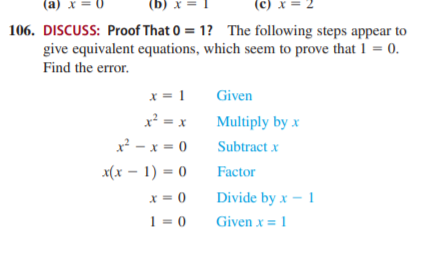# (a) x = 0 (b) x = (c) x = 106. DISCUSS: Proof That 0 = 1? The following steps appear to give equivalent equations, which seem to prove that 1 = 0. Find the error. x = 1 Given x² = x Multiply by x x² - x = 0 Subtract x x(x – 1) = 0 Factor X = 0 Divide by x – 1 1 = 0 Given x = 1

Question

DISCUSS: Proof That 0  1? The following steps appear to
give equivalent equations, which seem to prove that 1  0.
Find the error.help_outlineImage Transcriptionclose(a) x = 0 (b) x = (c) x = 106. DISCUSS: Proof That 0 = 1? The following steps appear to give equivalent equations, which seem to prove that 1 = 0. Find the error. x = 1 Given x² = x Multiply by x x² - x = 0 Subtract x x(x – 1) = 0 Factor X = 0 Divide by x – 1 1 = 0 Given x = 1 fullscreen

### Want to see this answer and more?

Experts are waiting 24/7 to provide step-by-step solutions in as fast as 30 minutes!*

*Response times may vary by subject and question complexity. Median response time is 34 minutes for paid subscribers and may be longer for promotional offers.
Tagged in
Math
Algebra

### Other